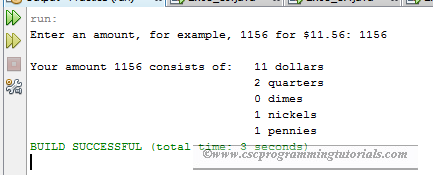The program below is the solution to Liang’s Introduction to Java Programming (9th Edition) Chapter 2 Exercise 2.24.

Question: Rewrite Listing 2.10, ComputeChange.java, to fix the possible loss of accuracy when converting a double value to an int value. Enter the input as an integer whose last two digits represent the cents. For example, the input 1156 represents 11 dollars and 56 cents.Program Output